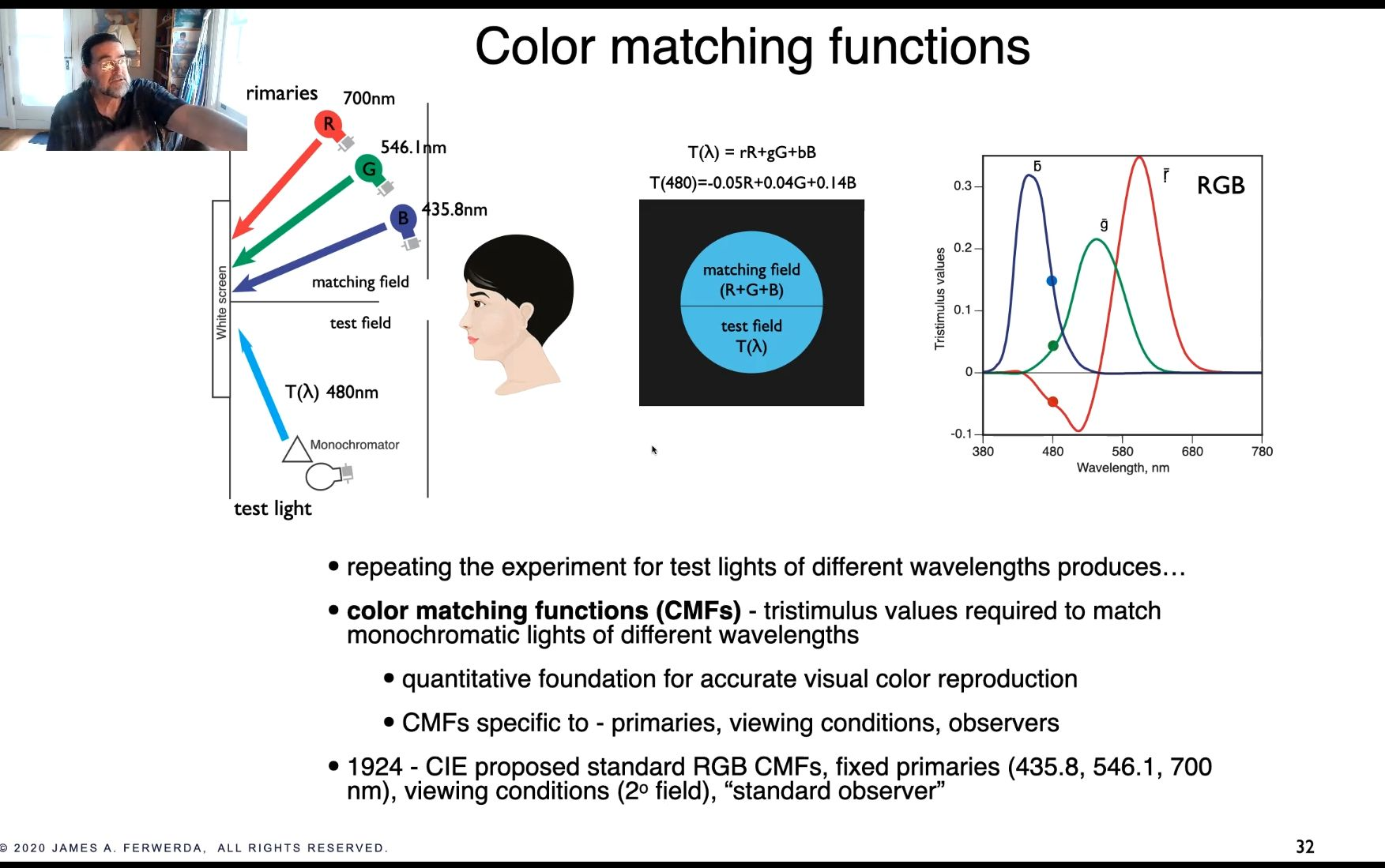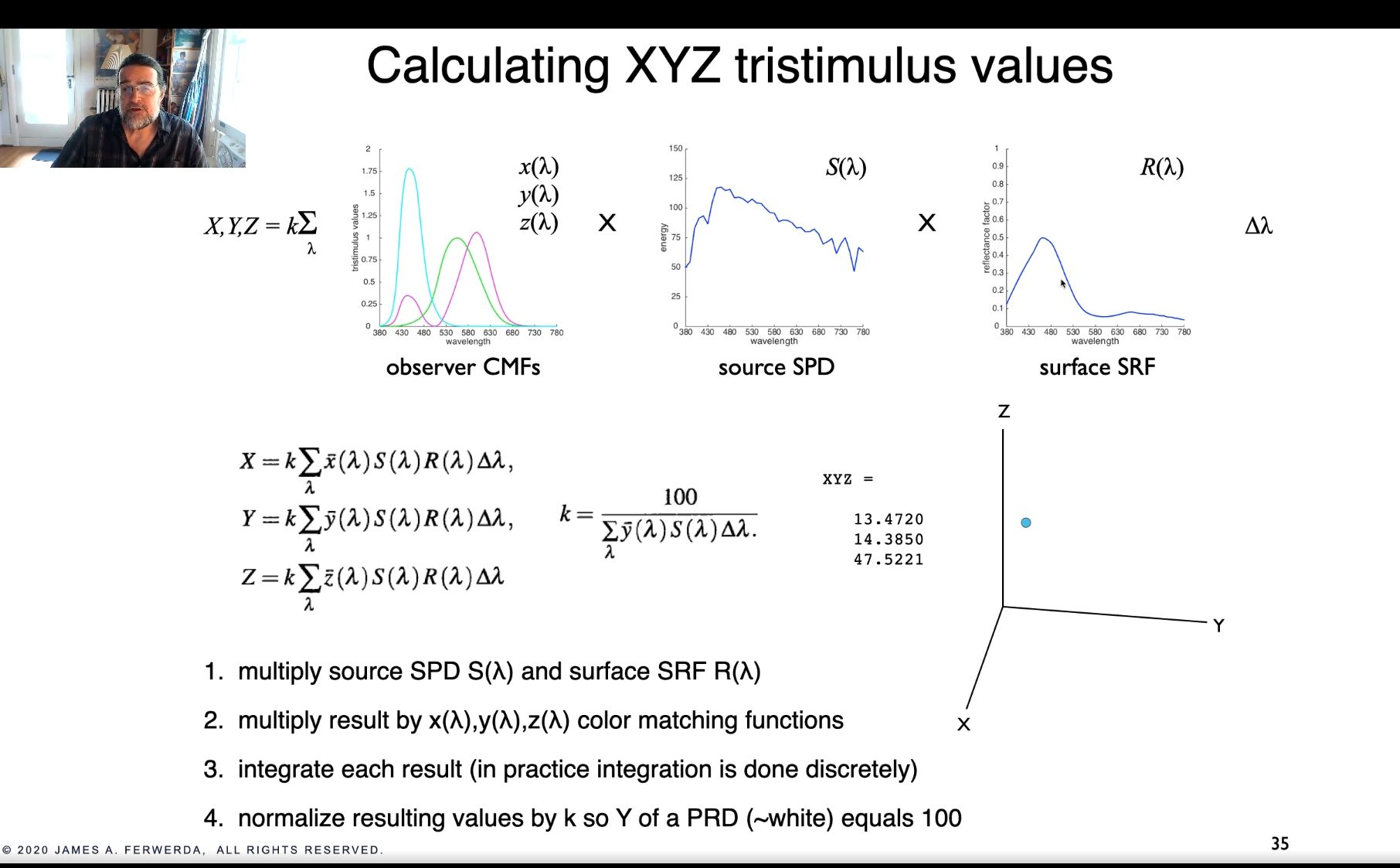Show Question

# CIE XYZ color matching curves

For a given wavelength $$\lambda$$ (x-axis), the 3 values of the X, Y and Z color matching functions represent the "amount" of the 3 hypothetical light sources that combine to produce a light that is perceived as being a pure light of wavelength $$\lambda$$.It's interesting to note that the lack of computers motivated the X, Y and Z functions to have the same integral area and for them to all be positive. This enabled easier calculations by humans.

The R, G and B color curves represent the same thing, but for physically realizable light. The values for the R, G and B function were determined by the below experiment.### Calculating XYZ values

You can calculate the XYZ values for some arbitrary light source if you know:

1. the spectral power distribution of this light source (the source SPD)
2. the surface spectral reflectance function (SRF)
3. the official XYZ function values#### Source

James Ferwerda, Siggraph 2020